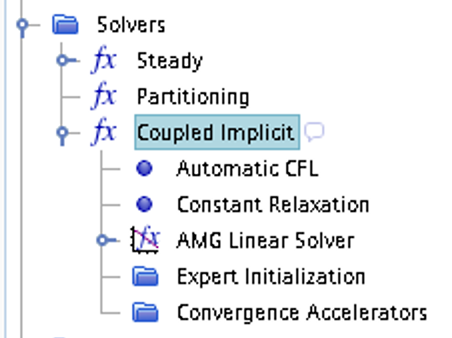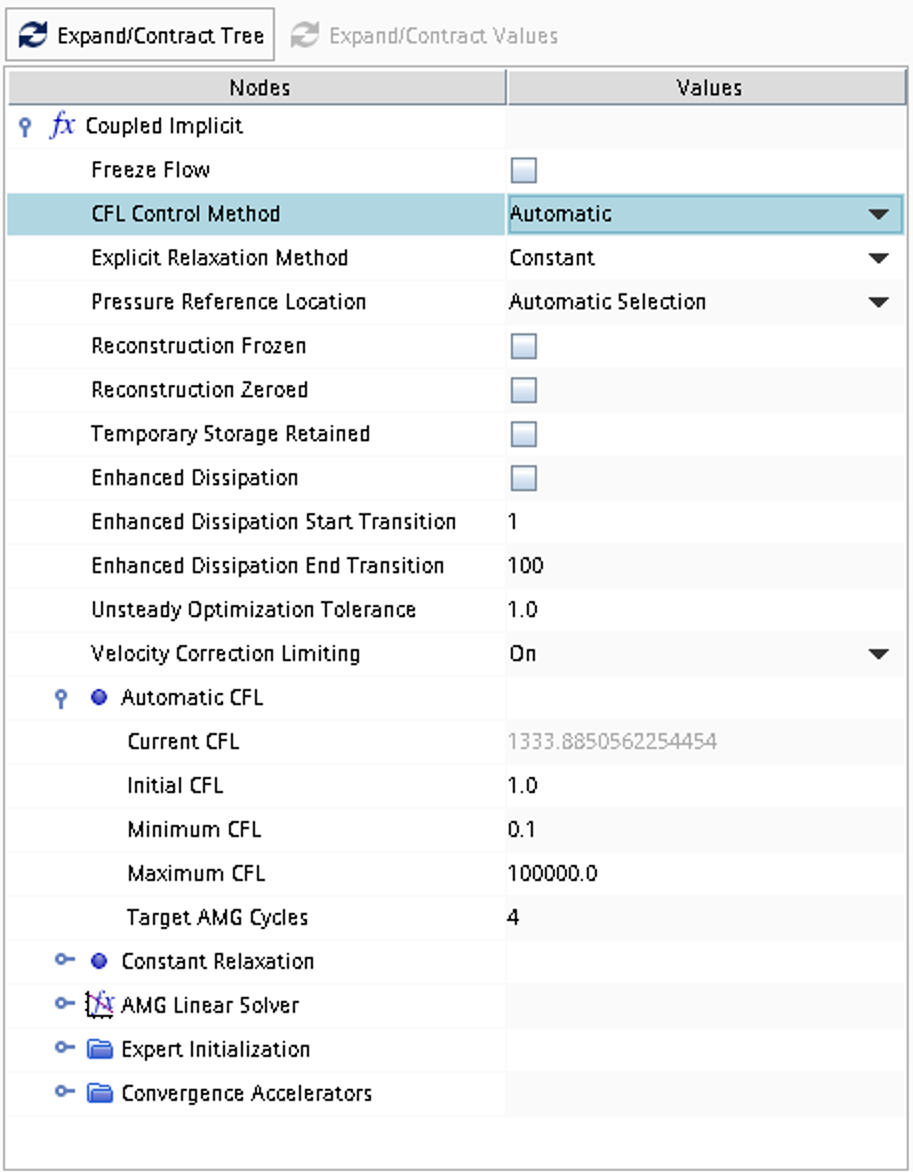## Case 6

Inviscid supersonic flow over a 2D compression/expansion ramp (steady-state)Inviscid compressible flow in two space dimensions. A geometry according to the picture below is defined and the problem consists of computing numerically the steady-state supersonic flow including the expected oblique shocks and expansion fan and compare it with the corresponding analytical solution.Figure 1: Overall view of computational domain, showing shape and extent of outer boundary

### Literature review

Suggested search topics

• Oblique shock
• Prandtl-Meyer expansion
• Shock-expansion theory
• Compression expansion ramp

### Specifications

• The compression/expansion ramp is symmetric
• $$x_2$$-$$x_1$$ = 0.5 m
• $$x_3$$-$$x_1$$ = 1.0 m
• For all operating conditions, ideal gas is assumed

For the specified operating conditions below, perform 2D inviscid simulations using STAR-CCM+ and compare with corresponding analytical solutions.

 operating condition 1 Mach number of incoming flow: 2.0 height of ramp: $$h$$=0.02 m
 operating condition 2 Mach number of incoming flow: 3.0 height of ramp: $$h$$=0.02 m
 operating condition 3 Mach number of incoming flow: 2.0 height of ramp: $$h$$=0.05 m
 operating condition 4 Mach number of incoming flow: 3.0 height of ramp: $$h$$=0.05 m

Find minimum value of $$h$$ that will result in a detached shock for an incoming flow with Mach number 2.0 and perform a simulation of that case. It might be a good idea to increase the value of $$h$$ slightly in order to make sure that the settings will result in a detached shock solution.

### Expected results and presentation

The flow field will contain oblique shocks and expansion fans. Although contour plots should be used for the visualization of these flow features it might be difficult to do a qualitative comparison of different simulations (simulations made using different meshes and numerical settings) and analytical results. Therefore, when comparing shock strength and location of flow features, it is recommended to extract data along an axial line somewhere above the ramp and compare the data in terms of $$xy$$-plots.

### Grid generation guidelines

There should not be any large jump in cell sizes anywhere. The changes in cell size must be smooth otherwise you might run into problems with convergence.

### CFD guidelines

Some general guidelines for the simulation:

• Don't forget to set the reference pressure to zero:
Continua -> Fluid -> Reference Values -> Reference Pressure
• In your project, you should investigate the performance of different numerical schemes. The numerical scheme is modified under Continua -> Fluid -> Models -> Coupled Flow. Note, in case you have not renamed the physics entry under Continua, the default name is Physics 1.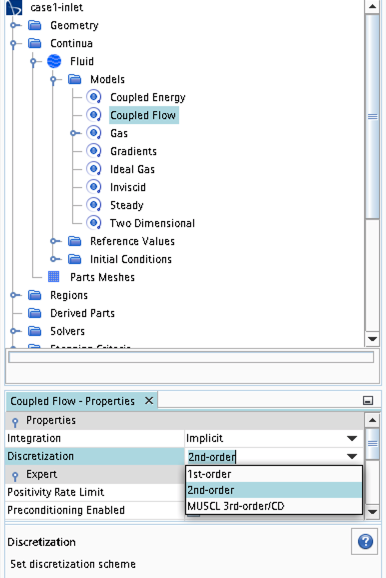• Some of the cases, or at least some of the operation conditions for some cases, will have convergence problems due to flow instabilities. In case you get that type of solver behavior, try to find another convergence criterion by for example measuring forces or massflow. You may also consider updating the mesh as it is crucial to resolve important flow features to reach convergence.
• Some of the cases should be simulated using a viscous solver. Choose Turbulent instead of Inviscid when you select models and then select an appropriate turbulence model.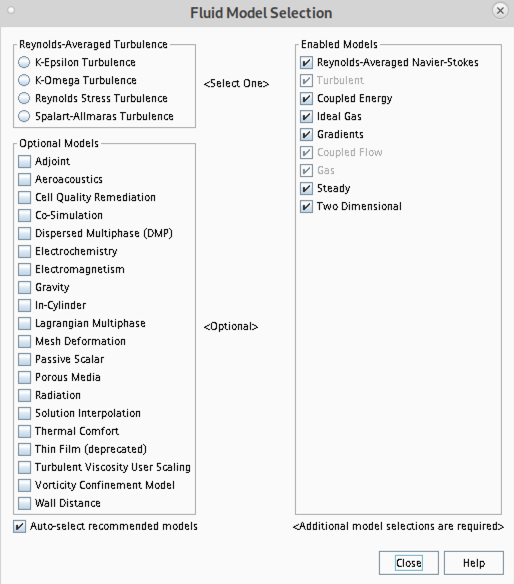• In case you would like to start your simulation from scratch, the flow field can be reset as indicated in the picture below (don't forget to initialize the flow field after the reset).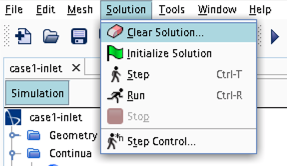• Time stepping approach (implicit/explicit) is selected under Continua -> Fluid -> Models -> Coupled Flow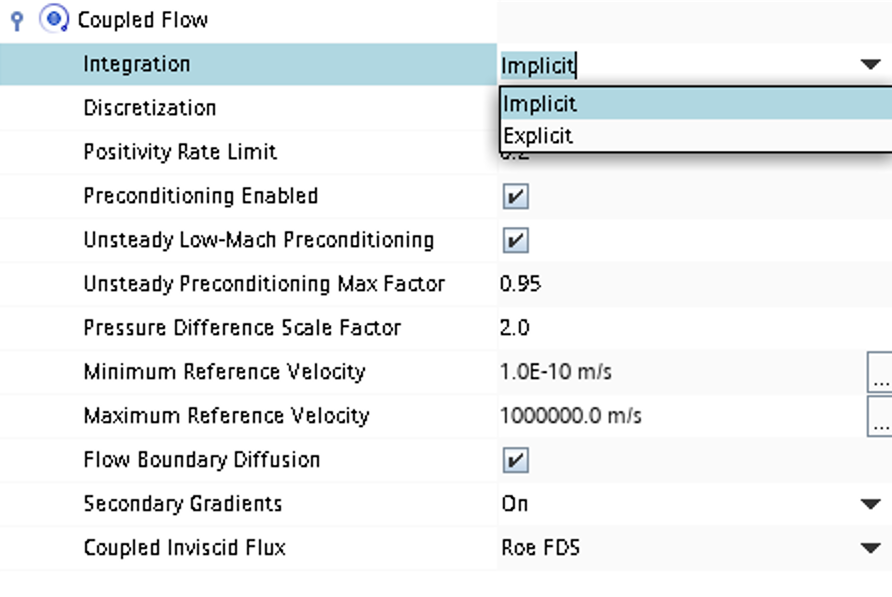• CFL number and other solver settings are found under Solver -> Coupled Implicit (or Solver -> Coupled Explicit)